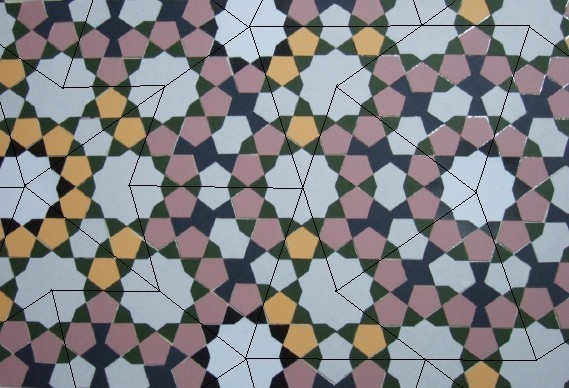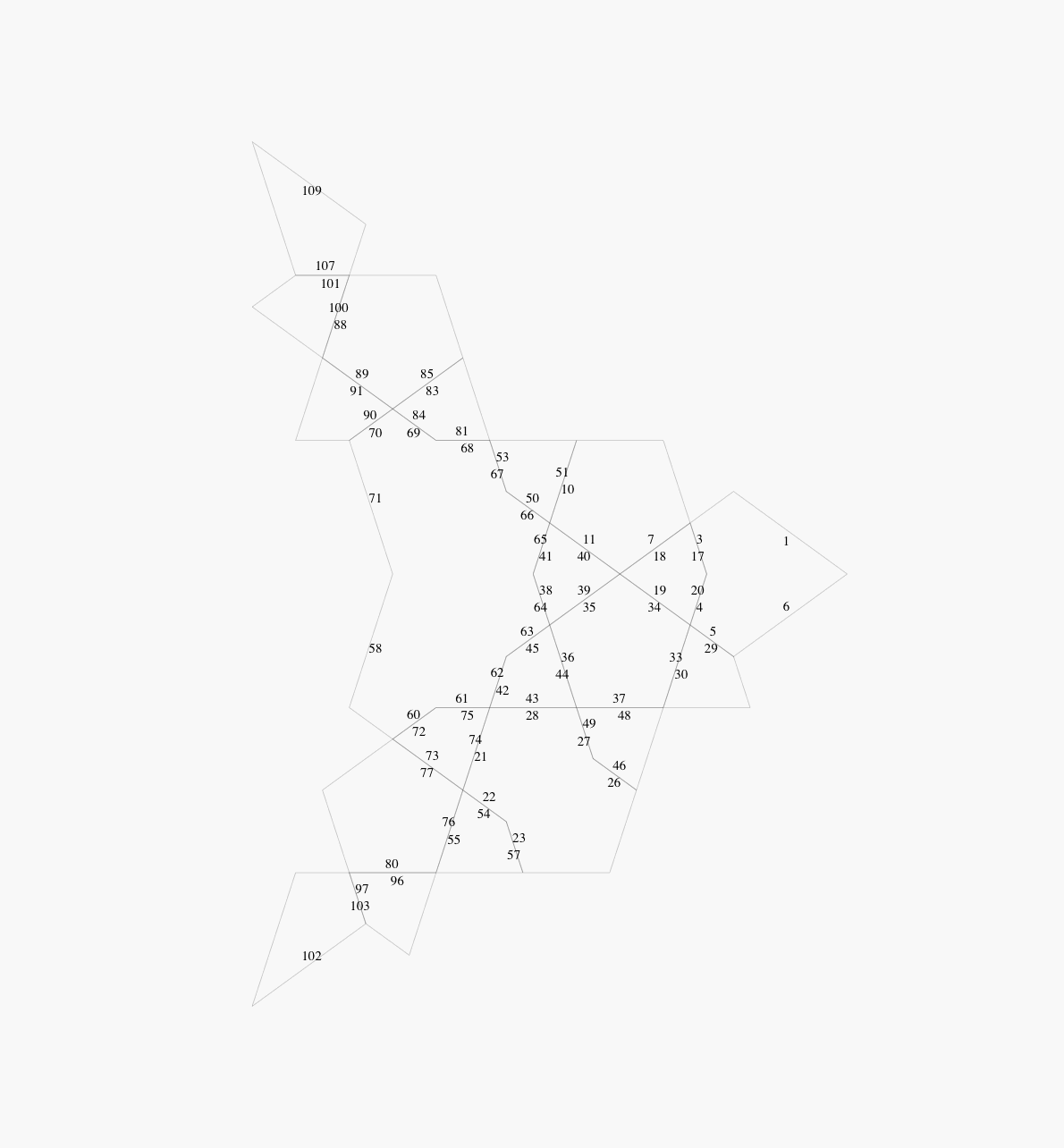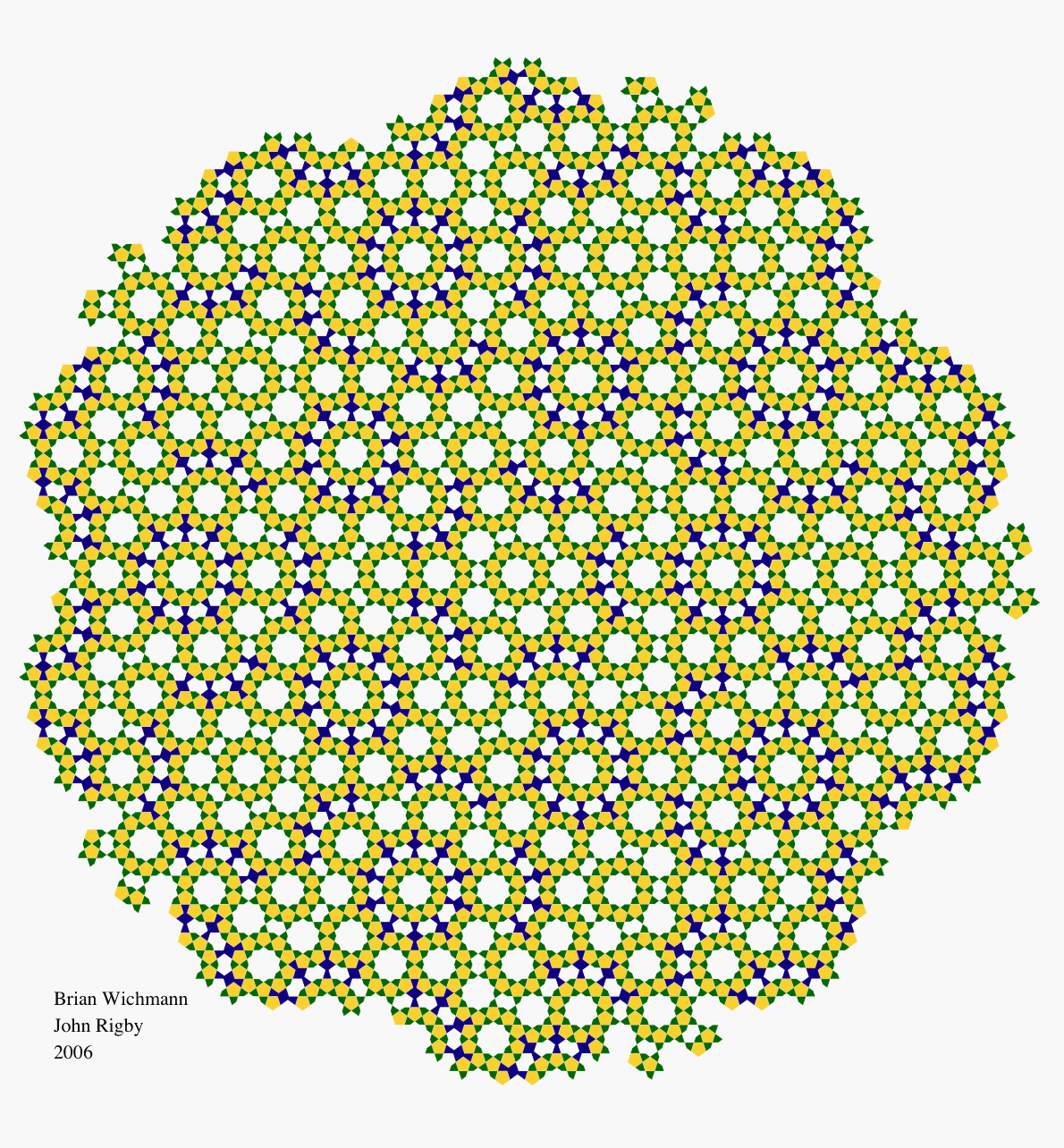# A Penrose-type Islamic Interlacing Pattern

## 1  Introduction

We first explain what is meant by a Penrose-type pattern, and we show how to create a Penrose-type interlacing pattern using just five shapes of tile - a pentagon, a 10-gon, an 8-gon that is always flanked by two attendant small kites to form a rhombus, and a star having ten small kites at its points (Figure 1). Then we describe how the computer drawings shown in this article were made.Figure 1: Basic shapes
The computer drawings, and the cardboard tiles photographed in Figures 1 and 4, were made by Brian Wichmann. The original patterns were created by John Rigby.
A Penrose tiling is a tiling of the plane by two types of tile, called kites and darts . The simple and obvious ways of fitting kites and darts together are precluded by simple rules governing the construction of Penrose tilings -kites and darts must only be fitted together in the approved manner, as described in . An example of part of a Penrose tiling is shown in Figure 2 (we explain the numbers added later). Penrose tilings are aperiodic and they have other properties described in .Figure 2: Penrose tiling from 
There are two ways (which can be combined) of creating a pattern using tiles - either we put together tiles of various shapes and sizes, each tile having a single colour, or we superimpose a partial pattern on a simple shape of tile - often a square but sometimes a rectangle or hexagon or other shape - then fit together copies of this simple tile; the partial patterns then fit together nicely (if they have been carefully designed) to create an overall pattern. We shall concentrate on the second method. To avoid confusion, it is important to distinguish between the pattern made up from all the superimposed partial patterns, and the pattern formed by the underlying tiles (a pattern of squares, rectangles, hexagons etc.)
Let us superimpose a partial pattern on the kites and darts of a Penrose tiling - the same pattern on each kite, and the same on each dart. When we fit together the kites and darts to form a Penrose tiling, the superimposed partial patterns form a basic Penrose-type pattern .
The superimposed pattern that we shall create here is itself a tiling - hence the possibility of confusion (mentioned earlier) between the tiling formed from the superimposed partial patterns and the pattern formed by the underling Penrose tiling! Our partial patterns are shown in Figure 3, and the Penrose tiling - or that part of it that we shall be using - in Figure 2. At each corner of a kite or dart in Figure 3, there is a sector of a star, and along each long edge there lies half a rhombus. When the patterned kites and darts are fitted together, complete stars and rhombuses are formed, as in Figure 4, and the final result is shown in Figures 9 and  10.Figure 3: A patterned kite and dartFigure 4: A basic Penrose-type pattern
We describe this pattern as an interlacing pattern because the edges between the small tiles can be replaced by a braid; at each vertex of the superimposed pattern two portions of braid cross each other, and these crossings can be made in an alternate over-and-under manner (Figure 11). The pattern is Islamic, or at least Islamic-style, because these shapes of tile occur frequently in Islamic patterns [2,4].
Ways of converting this pattern into more elaborate Penrose-type Islamic patterns are described in , Many periodic patterns created from the same shapes of tile can be found in .

## 2  Construction by computer

One method of constructing specific tiling patterns is to write a special program for the purpose. For a Penrose tiling, a simple recursive program can be used. (Sometimes the word `substitution' is used instead of recursion; but recursion is the computer technique used.) Such a program can only produce Penrose tilings, Here, we show how a more general program can be used. The program was originally designed to draw and catalogue more than 4,000 tilings in .
If we start with the existing program we find that there are three significant problems. Firstly, the program uses local connection rules to draw a tiling, but this will not work with a true Penrose tiling. Instead, only a local patch can be produced. However, the local patch can be big enough for our purposes, since if we start with a portion of tiling that is too large, this results in individual kites and darts which are too small to appreciate visually.
Another significant problem is that of rounding error. The drawing program places the individual tiles on the drawing area by matching edges. The matching needs to determine whether two points on the plane are `equal' given the inevitable rounding errors. Unfortunately, the rounding errors are bound to accumulate as the number of tiles grows. With over 6,000 tiles in the largest Penrose pattern that we have drawn, we must use double length floating point and calculate the basic tile data to that same precision.
The last significant problem is to handle the 6,000 tiles without having to specify in detail each tile. This is done by regarding each kite or dart as an individual `tile' even though they are themselves made up of tiles.
In Figures 5 to 8, the straight edges of the kite and dart in Figure 3 have been modified in such a way that the modified shapes fit together only in the approved manner (compare Figure 10.3.12 of ). The edge numbering is required in order to indicate how the edges fit together.Figure 5: Modified kite, showing edgesFigure 6: Modified kite in colourFigure 7: Modified dart, showing edgesFigure 8: Modified dart in colour
In the modified kite and dart we have got rid of the half-tiles along the edges, but it is still necessary to use sectors of stars at the vertices.
The strategy is to start with the recursively produced dart and kite pattern shown in Figure 10.3.20 of . This appears here as Figure 2, with the numbers of the individual kites and darts added. These numbers are used to determine how each kite and dart fits with its neighbours.
Note that the number of kites and darts required is reduced by factor of 5 due to the c5 symmetry. This reduces the work required substantially.
The final result of this work was two patterns (see Figures 9 and 10, the larger containing 6705 polygons - easily the largest I have produced.
Unfortunately, the division of the stars into pieces means that the program option to produce braiding will not work with these patterns. However, a large braided pattern is shown in Figure 11.Figure 9: Finished patternFigure 10: Extended patternFigure 11: Large braided pattern

## References


Branko. Grünbaum and G. C. Shephard, Tilings and Patterns, W. H. Freeman & Co., New York, NY, 1987.

John. Rigby, Creating Penrose-style Islamic Interlacing Patterns. Bridges Mathematical Connections in Art, Music and Science Conference Proceeding, 2006. Edited by R Sarhangi and J Sharp. pp.41-48.

Brian Wichmann, The World of Patterns, CD-ROM and booklet. World Scientific, 2001. ISBN 981-02-4619-6 URL

John Rigby and Brian Wichmann. Some patterns using specific tiles. Visual Mathematics. February 2006. URL

File translated from TEX by TTH, version 3.38.
On 10 Feb 2007, 11:19.